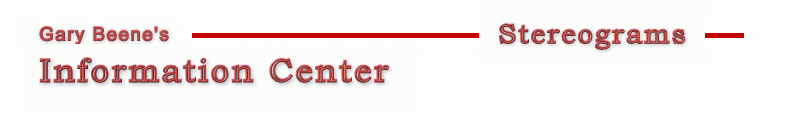Stereogram Algorithms - Oversampling This page shows how to modify the basic stereogram code to incorporate all of the algorithms discussed - smart links, hidden surface removal, and oversampling (I'm working on adding center-out processing - as soon as I figure it out!). ```1 Dim x As Long, y As Long, maxX As Long, maxY As Long, same() As Long 'basic 2 Dim z() As Single, mu As Single, E As Long, DPI As Long, s As Long 'basic 3 Dim right As Long, left As Long, w As Single 'basic 4 Dim L As Long 'smart links 5 Dim zt As Single, t As Long, visible As Long 'hidden surface removal 6 Dim R As Long, G As Long, B As Long, i As Long 'oversampling 7 Dim p() As Long, oversample As Long 'oversampling 8 oversample = 2: DPI = 72: E = 2.5 * DPI * oversample: mu = 0.2: w = 0.5 9 maxX = picDepth.ScaleWidth * oversample: maxY = picDepth.ScaleHeight 10 ReDim same(maxX), z(maxX, maxY), p(maxX, maxY): Randomize 11 For y = 0 To maxY 12 For x = 0 To maxX - 1 '1 scanline 13 z(x, y) = picDepth.Point(x / oversample, y) / 16777215 'initialize 14 same(x) = x 'initialize 15 s = (1 - mu * z(x, y)) * E / (2 - mu * z(x, y)) 'image plane separation 16 left = x - (s / 2) 'left link position 17 right = left + s 'right link position 18 If 0 <= left And right < maxX Then 19 t = 1 20 Do 21 zt = z(x, y) + 2 * (2 - mu * z(x, y)) * t / (mu * E) 22 visible = (z(x - t, y) < zt) And (z(x + t, y) < zt) 23 t = t + 1 24 Loop While visible And (zt < 1) 25 If visible Then 26 L = same(left) 'begin smart links code 27 While (L <> left) And (L <> right) ' 28 If L < right Then ' 29 left = L ' 30 L = same(left) ' 31 Else ' 32 same(left) = right ' 33 left = right ' 34 L = same(left) ' 35 right = L ' 36 End If ' 37 Wend 38 same(left) = right 'create link 39 End If 40 End If 41 Next x 42 For x = maxX - 1 To 0 Step -1 'set colors 43 If same(x) = x Then 44 p(x, y) = Int(Rnd + w) * vbWhite 'Rnd*vbwhite for color 45 Else 46 p(x, y) = p(same(x), y) 'link color 47 End If 48 Next x 49 Next y 50 For y = 0 To maxY: For x = 0 To maxX - 1 Step oversample 'average pixel colors 51 R = 0: G = 0: B = 0 52 For i = 0 To oversample - 1 53 R = R + p(x + i, y) Mod 256 54 G = G + (p(x + i, y) \ 256) Mod 256 55 B = B + (p(x + i, y) \ 256 \ 256) Mod 256 56 Next i 57 picOut.PSet (x / oversample, y), RGB(R / oversample, G / oversample, B / oversample) 58 Next x: Next y ```